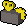## Towers

Towers puzzle scrolls are available from master clues in Treasure Trails. They can either be given by an NPC or from a scroll box. The objective is to place a tower in each square with a height between 1 and 5 so that no two towers in a row or column have the same height. The numbers around the edge tell you how many towers would be visible from that point, with taller towers obstructing the view of smaller towers behind them.

### General strategies

Some general strategies to solve these puzzles are listed below. It is advised to use pen and paper or any other way of writing necessary intermediate steps down. Start by writing all possible numbers (1 to 5) in each of the cells. We will eliminate possibilities now.

• If a column or row indicates 1, this means that the cell next to it has to be a 5.
• If a column or row indicates 1, and the # on the opposite end is also a 2 then the number next to the 2 MUST be a 4 as that is the only logical way to complete that row or column.
• If a column or row indicates 5, this means that the row or column will just be the numbers 1 through 5 in ascending order.
• If a column or row indicates 3, and the # on the opposite end is also a 3, then the center # on that column or row has to be 5.
• If a column or row indicates 4, this means that
• The column or row CANNOT have the numbers 3, 4 or 5 in the first cell. Otherwise you can never see 4 towers. The only remaining possibilities for the first cell are thus 1 and 2.
• The column or row CANNOT have the numbers 4 or 5 in the second cell. Otherwise you can never see 4 towers again. The only remaining possibilities for the second cell are thus 1, 2 and 3.
• The column or row CANNOT have the number 5 in the third cell. Otherwise you can never see 4 towers again. The only remaining possibilities for the third cell are thus 1, 2, 3 and 4.
• If a column or row indicates 3, this means that
• The column or row CANNOT have the numbers 4 or 5 in the first cell. Otherwise you can never see 3 towers. The only remaining possibilities for the first cell are thus 1, 2 and 3.
• The column or row CANNOT have the number 5 in the second cell. Otherwise you can never see 3 towers again. The only remaining possibilities for the second cell are thus 1, 2, 3 and 4.
• If a column or row indicates 2, this means that
• The column or row CANNOT have the numbers 5 in the first cell. Otherwise you can never see 2 towers. The only remaining possibilities for the first cell are thus 1, 2, 3 and 4.
• The column or row CANNOT have the number 4 in the second cell. Otherwise you will always see 3 towers. The only remaining possibilities for the second cell are thus 1, 2, 3 and 5.
• After the first elimination of possibilities and possibly writing down some 5's, we need to do the following:
• Look in rows and columns to check if a certain number only appears once. This number can only be put there.
• Since each number only appears once in a row or column, eliminate the number from the possibilities of other cells in the same row and column.
• Repeat the above two steps.
• The remainder should leave you with something you can solve yourself by simple logic. Mostly, there will be multiple possibilities: pick one free choice and fill in the rest.

### Automated solverThis is a dynamic calculator that requires Javascript.This takes user input via Javascript, and may use changing prices from the Grand Exchange Market Watch.If prices appear to be outdated, purge the page by clicking here.

This calculator will show all possible combinations for a given input. All inputs accept a value between 1 and 5, when all fields are filled in press solve. Then choose one of the solutions and copy the numbers to Runescape.

Tip: use the Tab key to easily jump to the next input field.

 name=in12|type=int|value=""|range=1,5|entersublist=calc|style=max-width:35px name=in13|type=int|value=""|range=1,5|entersublist=calc|style=max-width:35px name=in14|type=int|value=""|range=1,5|entersublist=calc|style=max-width:35px name=in15|type=int|value=""|range=1,5|entersublist=calc|style=max-width:35px name=in16|type=int|value=""|range=1,5|entersublist=calc|style=max-width:35px name=in21|type=int|value=""|range=1,5|entersublist=calc|style=max-width:35px name=in27|type=int|value=""|range=1,5|entersublist=calc|style=max-width:35px name=in31|type=int|value=""|range=1,5|entersublist=calc|style=max-width:35px name=in37|type=int|value=""|range=1,5|entersublist=calc|style=max-width:35px name=in41|type=int|value=""|range=1,5|entersublist=calc|style=max-width:35px name=in47|type=int|value=""|range=1,5|entersublist=calc|style=max-width:35px name=in51|type=int|value=""|range=1,5|entersublist=calc|style=max-width:35px name=in57|type=int|value=""|range=1,5|entersublist=calc|style=max-width:35px name=in61|type=int|value=""|range=1,5|entersublist=calc|style=max-width:35px name=in67|type=int|value=""|range=1,5|entersublist=calc|style=max-width:35px name=in72|type=int|value=""|range=1,5|entersublist=calc|style=max-width:35px name=in73|type=int|value=""|range=1,5|entersublist=calc|style=max-width:35px name=in74|type=int|value=""|range=1,5|entersublist=calc|style=max-width:35px name=in75|type=int|value=""|range=1,5|entersublist=calc|style=max-width:35px name=in76|type=int|value=""|range=1,5|entersublist=calc|style=max-width:35px name=buttoncalc|type=button|value=Solve!|sublist=calc name=buttonreset|type=button|value=Reset|sublist=reset
name=calcout|type=output
calc|

let(buttoncalc, "Solving...") parse(calcout, "{"+"{Calculator:Towers solver/template |in12 = " + in12 + " |in13 = " + in13 + " |in14 = " + in14 + " |in15 = " + in15 + " |in16 = " + in16 + " |in72 = " + in72 + " |in73 = " + in73 + " |in74 = " + in74 + " |in75 = " + in75 + " |in76 = " + in76 + " |in21 = " + in21 + " |in31 = " + in31 + " |in41 = " + in41 + " |in51 = " + in51 + " |in61 = " + in61 + " |in27 = " + in27 + " |in37 = " + in37 + " |in47 = " + in47 + " |in57 = " + in57 + " |in67 = " + in67 + "}}") let(buttoncalc, "Solve!")

reset|

let(calcout, "") let(in12, "") let(in13, "") let(in14, "") let(in15, "") let(in16, "") let(in72, "") let(in73, "") let(in74, "") let(in75, "") let(in76, "") let(in21, "") let(in31, "") let(in41, "") let(in51, "") let(in61, "") let(in27, "") let(in37, "") let(in47, "") let(in57, "") let(in67, "")